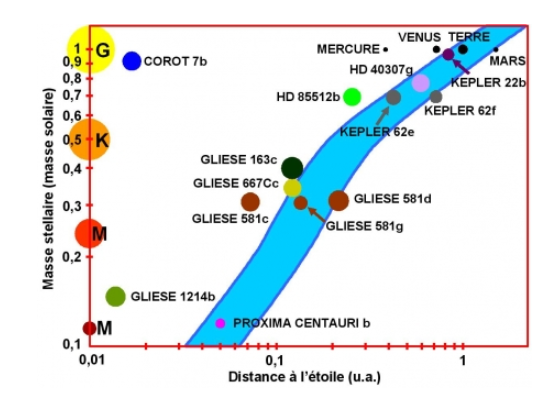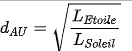# AXdlv

Members

6

## Posts posted by AXdlv

### Habitable zone of a star

8 minutes ago, Moontanman said:

Ok, thanks for the clarification, I have been following Sara Seager, who works for NASA and her take on the habitable zone is a bit more complex. Also it should be noted that the Earth with no atmosphere would have an average temp below freezing.

Thank you for the name. I knew Sara Seager for her "Drake equation". Is it this calculation ? https://arxiv.org/pdf/1304.3714.pdf

### Habitable zone of a star

31 minutes ago, Moontanman said:

Do these calculations assume a planet identical to Earth?

According to my research, I saw that the size of the planet is not very important. But obviously, the mass has an impact on the presence of an atmosphere, so on the temperature.
But I just wanted to know how the habitable zone was calculated, this zone says more about the star than on the planet, as it was said before.

### Habitable zone of a star

14 hours ago, Sensei said:

3.828*10^26 W / ( 4 * PI * ( 150*10^9 * 0.95 )^2 ) = 1500 W/m^2 (0.95 AU).

So inner edge is at least "right"..

It is several times mentioned on Wikipedia, in "Solar System estimates" section:

I picked up +- 10 % of power tolerance as an example values, which are acceptable for human.

in "Solar System estimates" section, we can see there is no general consensus between scientists, how to estimate it. They make predictions which require e.g. special non-Earth-like atmospheres, with significantly different pressures.

I would like to fix it a bit. The majority of Carbon-based living organisms will be dead at temperatures above 46 C, because their proteins will be denatured..

OK thanks a lot! So, if I understood correctly, in our solar system, we did not define the HZ. So, this representation is correct ?In fact, I'd like to do a program that says (with the mass of the star, is luminosity and the distance between the planet and the star using Kepler's law) if the planet is or isn't in the habitable zone... So if I understood I must take our actuel delimitation for the solar system and transpose it to another system.

(3,8*10^26)/4*PI*(0,95*1,49*10^11)^2 = 1500 W.m^-2

(3,8*10^26)/4*PI*(2,4*1,49*10^11)^2 = 236 W.m^-2

And I take Kepler 452 with P = 1,2 * 3,8 * 10^26 W

Outer : ((1,2*3,8*1026 )/(4*PI*236))1/2 = 3,9 * 1011 m

Inner : ((1,2*3,8*1026 )/(4*PI*1500))1/2 = 1,55 * 1011 m

For Kepler from 1,55*10^11 to 3,9 * 10^11 m is "habitable" ?

### Habitable zone of a star

2 hours ago, Sensei said:

I would define "habitable zone" as distance from the star in which planet is receiving 1370 Watts per square mater of surface +- couple (or couple dozen) percent tolerance.

1370 W/m^2 is measured radiation of the Sun, and by applying reverse of inverse-square law, we can calculate total power of the Sun.

P=P04πr2

P0=P4πr2

P0=137043.14159265(150109)2=3.81026W

So, if we will use +-10% tolerance, star must deliver (approximately) 1200 W/m^2....1500 W/m^2.

You should be able to calculate min and max radii for this range by yourself now.

Carbon-based organic life requires water. Water must be able to exist in liquid form. Which is in temperature range 0 ... 100 C (at standard pressure). Too large radiation from the star, and water will vaporize, like on Venus. Too low radiation, and it'll freeze.

There are existing super cold stars which emit fraction of energy which is emitted by the Sun. So distance between such super cold star and planet would have to be very small. e.g. star which has 1% of power of the Sun, must have planet located at distance ~15 mln km.

Thank you for this calculation ! In the solar system, the habitable zone is between 0.95 UA and 2 AU. I do not find these values if I use your calculation ... Is it because the Earth is not in the middle of this zone ?

What are the minimum and maximum "P" for a temperature between 0 ° C and 100 ° C? (I mean Pmin=1200 W/m^2 and Pmax=1500W/m^2 ? or is it another value)

### Habitable zone of a star

17 hours ago, Moontanman said:

I'm not sure how you are defining habitable zone, in our solar system both Venus and Mars are within the habitable zone but both have atmospheres that prohibit life.

I know this is not an answer but I want to see what others have to say and I'll do a bit of digging too..

I think (but I'm not sure) that the albedo (so the composition of the atmosphere) is not taken into account to define the habitable zone. But I can be wrong...

### Habitable zone of a star

Hi !

I need your help to find a formula to calculate the habitable zone of a star ... I searched and searched without having the answer ...

I found this calculation:But it just defines a radius. I would like to calculate the lower limit of the ZH and its upper limit. I found other calculations with Boltzman's law, but I can not find results that work.

So if you have an idea I'd be happyThanks (sorry for my english)

×